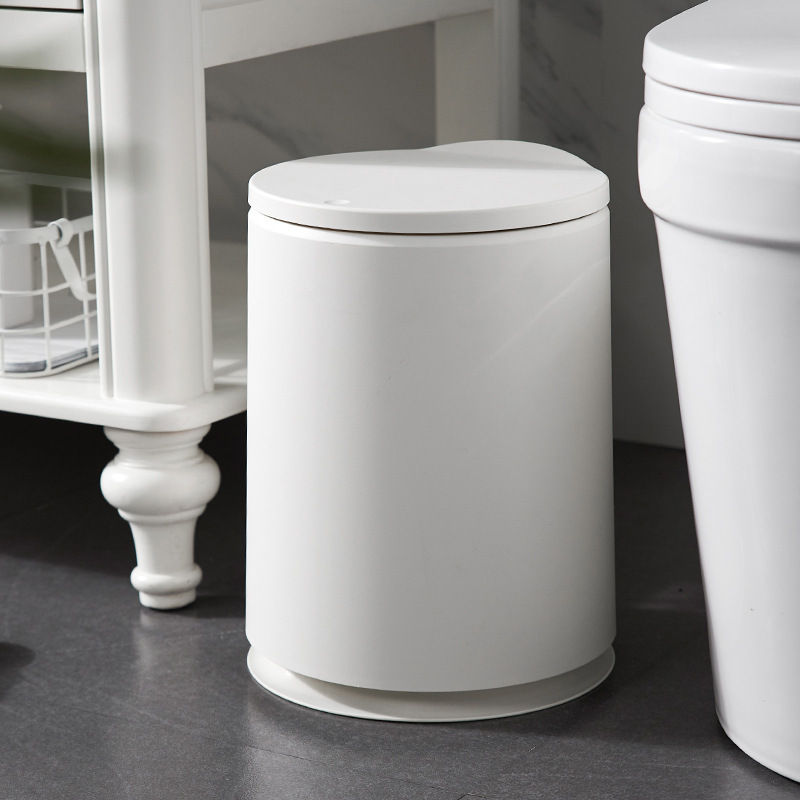#F u l l H o u s e R o u n d P r e s s T r a s h F a m i l y L i v i n g R o o m W i t h C o v e r e d S a n i t a r y B u c k e t C r e a t i v e T o i l e t P a p e r B a s k e t

Updated Wednesday 16-Oct-2019## F u l l H o u s e R o u n d P r e s s T r a s h F a m i l y L i v i n g R o o m W i t h C o v e r e d S a n i t a r y B u c k e t C r e a t i v e T o i l e t P a p e r B a s k e t 3

THB 327.86